×
Get Full Access to MSU - CSE 6613 - Class Notes - Week 7
Get Full Access to MSU - CSE 6613 - Class Notes - Week 7

×

MSU / Engineering / SO 4613 / How to store a matrix into a program?

# How to store a matrix into a program? Description

##### Description: All examples worked on class on how to operate with dictionary and lists and loops on Python. Detailed information and step per step on each code.
9 Pages 46 Views 1 Unlocks
Reviews

September 19, 2016

## How to store a matrix into a program?WORKING WITH MATRIX

1) Suppose you want to store a matrix into a program. So basically you create a list of lists.  2) Ask to print a number in a specific location of a matrix. So, matrix [row] [column]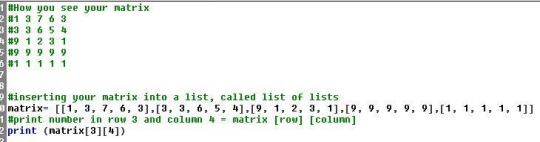It will print 9 on the keyboard.

3) The problem happens when you do a matrix with a bunch of zeros. It is called sparse  matrix, matrix with lots of zeros. The problem is that if you need to do many calculations  you will end up with more zeros.

## What happens when you do a matrix with a bunch of zeros?The problem it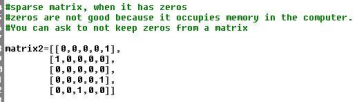Don't forget about the age old question of Why does sex categorization matter?
If you want to learn more check out What are the four basic blood types?

occupies memory

because in this case it

will store 24 numbers

in which only 4

matters. Because

zeros are irrelevant.

Matrixes are important in molecular biology because you can store a matrix with sequence  similarities, like the ones produced by Clustal Omega.

4) Trying to find a number on the matrix from your keyboard.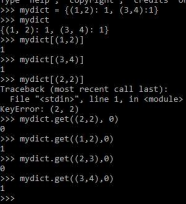## Why does matrix be important in molecular biology?So you can tell that same matrix above you have 1 in the

positions (1,0), (4,2) and (0,4). And put these positions on a

dictionary.

So, dict = {(1,0):1, (4,2):1, (0,4):1}

That means that (row:col) has 1.

Then you can ask what number it is on:

Dict = (row, col)

However, because you did not define what numbers you have  We also discuss several other topics like What is the formula for kinematics?

on other positions at first it will give you an error.

You can use dict.get ((row,col), number) where number

turns to be the missing number you want it to be. So it this

case I want that the positions I didn’t insert a number become  If you want to learn more check out What are archaea called extremophiles?

zero and so all other positions, using same code, if they had a

number described before, they still have same number (1).

Dic.get = let you treat a dictionary as a matrix.

So number can be a number or a string. It does not matter.

Same thing can be done on your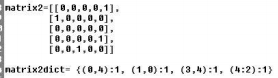interpreter. So there, you can turn

your matrix in a dictionary and just

input where the numbers that are not

zero are.

Dictionary.get((row,col), number)

can also be use on your interpreter.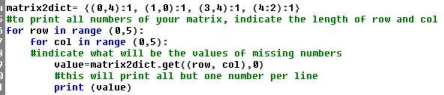Don't forget about the age old question of What is a measure of how much consumers and producers will react to changes in the market?

Doing the same code, but asking to print the numbers on the same line.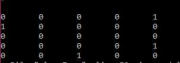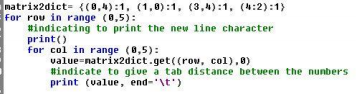Turning your matrix in a dictionary is a good way to save memory on your computer. Don't forget about the age old question of What is the meaning of recombinant dna?

……………………………………………………………………………………………………

*** When you have a list you can use codes to work on the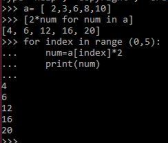list of comprehension. For example, imagine you have a list

and you want to multiply all your numbers from your list by

2.

You can do the same thing using a loop, “for num in a:

num*2…”

If you want a list of that, you just append the number.

If you do a*2 instead of a[index]*2, instead of multipliying each number from a, it will make 2  copies of a. .................................………………………………………………………

Build a matrix.

When you write matrix = [0*5], it means that you have a matrix with 5 zeros in one line. When you write matrix = [[0*5]*5], it means you have a matrix with 5 zeros in 5 lines.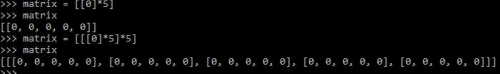WORKING ON MATRIX OF GENE EXPRESSION

Imagine you have a matrix where each line represents a single gene and each column gives you  expression of different samples from that same gene. You input file will look like this: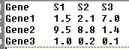So, first same steps as always: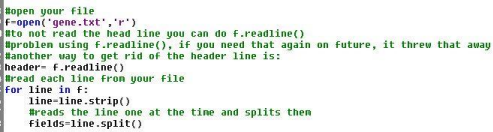Imagine you can take the average of these numbers and print it in a dictionary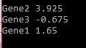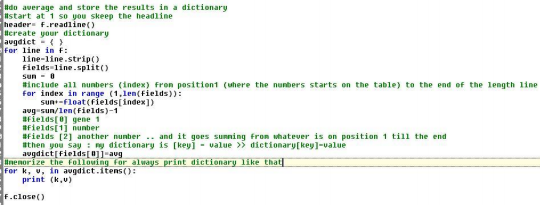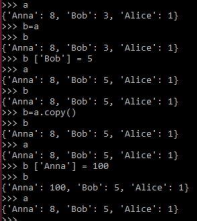**Dictionary can be treated as lists, with same

commands. For example, if you have 2 dictionaries

and one is equal to the other one and you change one,

because both have same ID, the other one will change

too.

So if you want to change one without affect the other

you need to save copy.

September 21, 2016

**Observations about assignment 4 – working on lists.

When you create lots of sequences, you cannot relate the lists opening each one. For example: Names= [‘a’, ‘b’ , ‘c’]

Ids = [40, 60, 80]

Use:

For index in range (0, len(names))

Print (names[index] + ids[index])

Don’t do it:

For name in names OR for id in ids: because it has no way to relate both lists as they were associated to each  other.

WORKING ON DICTIONARY – EXAMPLE

1) Imagine you want to create a dictionary where you will store gene names and sequences that must be  inserted from the keyboard.

In this dictionary of tuples, key will be the gene name and the value the sequence.

Make sure the no repeated gene name or sequence occurs.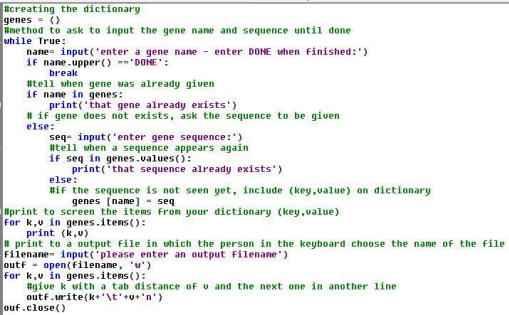WORKING ON FASTA FILES

1) Fasta have a particular way to save the gene sequences, in which the same sequence is break into  multiple lines you it can make it easier to the user see a long sequence. For example:

2) Imagine you want to compute the GC content for each sequence.

b. Read one line one at the time

c. Print only the gene names. This is important to know how many genes you have and who are they. d. If you want to count the number of genes you could do just “count+=1”

e. Printing the entire sequence with the gene name:

Another way:

The best way, by using dictionary:

Remember, the order of your genes will always change.

f. Printing the GC content

g. Printing the GC content in an out file:

h. Printing your dictionary keys in order.

Need to turn it into a list first!

Thinks that might be on the test:

1) Suppose a dictionary exists that contains GC content for many different genes, with gene name  for the key. Print the GC content for the gene1.

Note the dictionary was already made. The content was already calculated. Give just the code  to print! Only the print code.

Print (gcdict[‘gene1’])

2) Suppose everything from the first question was already done, replace the value of Gene 1 to  0.5.

gcdict[‘Gene1’] = 0.5

3) Given the genes bellow that were in the worked dictionary from the first question, give me all  genes with a GC content of 0.5.

Gene1 0.5

Gene2 0.1

Gene3 0.3

Gene4 0.5

Gene5 0.1

Gene6 0.7

For k, v in gcdict.items( ):

If v==0.5:

Print(k)

4) From the question above. If a code is given to print the genes with GC content of 0.1, how the  keyboard would print it?

(gene2, gene5)

5) What about GC content of 0.7?

(gene6, )

***don’t forget the comma!!!

Page ExpiredIt looks like your free minutes have expired! Lucky for you we have all the content you need, just sign up here